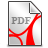# Open arity poses a problem in predicate logics

A closer look at mathematical proofs led Gottlob Frege to realize that Aristotle's syllogism logic was not sufficient for many theorems. He developed what today is called first-order predicate logic. It is usually thought that predicate logic is sufficient for the theory of natural numbers. However, this first step of modern logic development again is not sufficient. One needs another step, especially to allow for so-called open arity of arrays. This second step cannot be done in general in object-language based on predicate logic but only by metalanguage. Therefore one needs something like the FUME-method (put forward by the author) which allows for a precise treatment of both language levels. Dot-dot-dot … is not admissible in predicate logics as it needs some kind of recursion. In metalanguage, however, one has to introduce some basic recursion right from the setup (but it is much weaker than primitive recursion).

For natural numbers two examples are given, one for a concrete version of  Robinson arithmetic and one for recursive arithmetic. Without the second step to metalanguage one cannot express some of the most important so-called theorems of number theory in a direct fashion, leave alone prove them. Actually some are not theorems but metatheorems. The examples comprise Chinese remainders, Gödel's beta-function, little Gauss's summing up of numbers, Euclid's unlimited primes and the canonical representation of a natural number (fundamental theorem of natural arithmetic).

After one has included the second step which allows one to talk about open arities in metalanguage one can tackle the problem of talking about number-arrays in object language. One can do this to a certain extent by coding number-arrays by (usually) two numbers. This can be done even in Robinson arithmetic using 'Gödel's beta-function'. But one has to make use of  the second step before one can return to object-language. Of course, the introduction of two tiers, i.e. object-language and metalanguage, is necessary for many other areas of mathematics, if not to say, most of them.

Download OpenAri1.0.pdf  'Number Theory beyond Frege - On the necessity of open arity', version 1.0 (13 pages) , first publication for priority 23.11.2019 viXra: 1911.0390v1OpenAri1.1.pdf (508.85KB)OpenAri1.1.pdf (508.85KB)